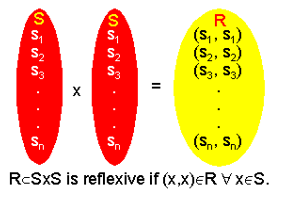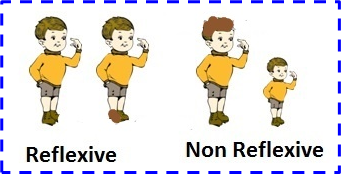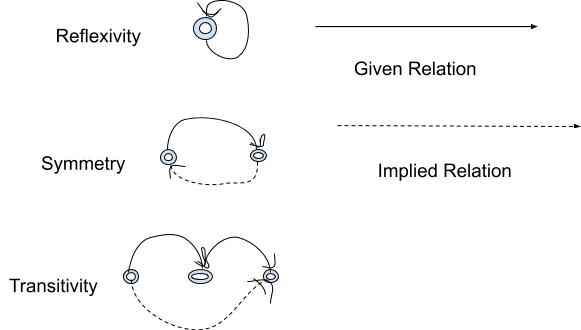Mathematics

# What do you mean by a Reflexive Relation?

3k views

### Table of Contents

 1 Introduction 2 What is a Reflexive Relation? 3 How to prove a relation is reflexive? 4 Reflexive Relation Example 5 Irreflexive 6 Summary 7 FAQs 8 External References

29 October 2020

Read time: 5 minutes

## Introduction

### What is a relation?

A relation exists between two things if there is some definable connection in between them.

For example, Father, Mother, and Child is a relation, Husband and wife is a relation, Teacher & Student is a relation.

Every relation has a pattern or property. Also, every relation involves a minimum of two identities.

Relations between sets do not only exist in mathematics but also in everyday life around us such as the relation between a company and its telephone numbers

## Downloadable PDF

Here are some additional points that talk about reflexive relations. To view them click on the Download button.

 📥 What do you mean by a Reflexive Relation? Download

## What is a Reflexive Relation?

A relation is said to be a reflexive relation on a given set if each element of the set is related to itself.

Let us take a relation R in a set A

It is proven to be reflexive, if (a, a) ∈ R, for every a∈ AReflexive relation example:

Let’s take any set K =(2,8,9}

If Relation M ={(2,2), (8,8),(9,9), ……….} exists, then relation M is called a Reflexive relation.If we really think about it, a relation defined upon “is equal to” on the set of real numbers is a reflexive relation example since every real number comes out equal to itself.

While using a reflexive relation, it is said to have the reflexive property and it is said to possess reflexivity.

An equivalence set requires all properties to exist among symmetry, transitivity, and reflexivity.

### Reflexive Relation Table

Here is a table of statements used with reflexive relation which is essential while using reflexive property.

Statement  Symbol
"is equal to" (equality)  =
"is a subset of" (set membership)  ⊆
"divides" (divisibility)  ÷ or /
"is greater than or equal to"  ≥
"is less than or equal to"  ≤

### Reflexive Relation Formula

The number of reflexive relations on a set with ‘n’ number of elements is given by;

\boxed{\begin{align}N=2^{n(n-1)}\end{align}}

Where N = total number of reflexive relation

n = number of elements

Suppose, a relation has ordered pairs (a,b). Here the element ‘a’ can be chosen in ‘n’ ways and the same for element ‘b’. So, the set of ordered pairs comprises pairs.

As per the definition of reflexive relation, (a, a) must be included in these ordered pairs.

On observing, a total of n pairs will exist (a, a). Hence, the number of ordered pairs here will be n2-n pairs.

Therefore, the total number of reflexive relations here is $$2^{n(n-1)}$$.

## How to prove Reflexive Relation?

As discussed above, the Reflexive relation on a set is a binary element if each element of the set is related to itself.

### When is a relation reflexive?

Let X be a set and R be the relation property defined in it.

R is set to be reflexive if (x, x) ∈ R for all x ∈ X that is, every element of X is R-related to itself, in other words, xRx for every x ∈ X.

A relation R in a set X is not reflexive if at least one element exists such that x ∈ X such and (x, x) ∉ R.

For example, taking a set X = {p, q, r, s}.

The relation R11 = {(p, p), (p, r), (q, q), (r, r), (r, s), (s, s)} in X follows the reflexive property, since every element in X is R11-related to itself.But the relation R22 = {(p, p), (p, r), (q, r), (q, s), (r, s)} does not follow the reflexive property in X since q, r, s ∈ X but (q, q) ∉ R22, (r, r) ∉ R22 and (s, s) ∉ R2

## Reflexive Relation Examples

Reflexive Questions

Q.1: A relation R is on set A (set of all integers) is defined by “x R y if and only if 2x + 3y is divisible by 5”, for all x, y ∈ A. Check if R follows reflexive property and is a reflexive relation on A.

Solution:

Let us consider x ∈ A.

Now 2x + 3x = 5x, which is divisible by 5.

Therefore, xRx holds for all ‘x’ in A

Hence, R is a reflexive relation.

Q.2: A relation R is defined on the set of all real numbers N by ‘a R b’ if and only if |a-b| ≤ b, for a, b ∈ N. Show that the R is not a reflexive relation.

Solution:

The relation won’t be a reflexive relation if a = -2 ∈ R

But |a – a| = 0 which is not less than -2(= a).

Therefore, the relation R is not reflexive.

Q.3: Consider a relation R on the set A given as “x R y if x – y is divisible by 5” for x, y ∈ A. How to prove a relation is reflexive?

Solution:

Let us consider, x ∈ A.

Then x – x is divisible by 5.

Since x R x holds for all x in A

Therefore, R is a reflexive relation.

Q.4: Consider the set A in which a relation R is defined by ‘x R y if and only if x + 3y is divisible by 4, for x, y ∈ A. Show that R follows the reflexive property and is a reflexive relation on set A.

Solution:

Let us consider x ∈ A.

So, x + 3x = 4x, is divisible by 4.

Since this x R x holds for all x appearing in A.

Therefore, R is a reflexive relation.

## Irreflexive relation

R on a set X is called a irreflexive relation if no (x,x) € R holds for every element x € X.i.e. if set X = {x,y} then R = {(x,y), (y,x)} is an irreflexive relation.

### Irreflexive Relations on a set with n elements

A relation has ordered pairs (x,y). For Irreflexive relation, no (x, x) holds for every element a in R. It is also defined as the opposite of a reflexive relation.

Now for any Irreflexive relation, the pair (x, x) should not be present which actually means total n pairs of (x, x) are not present in R, So the number of ordered pairs will be n2-n pairs.
So the total number of reflexive relations is equal to $$2^{n(n-1)}$$

## Summary

Set theory is seen as an intellectual foundation on which almost all mathematical theories can be derived

Reflexive relation is an important concept to know for functions and relations.

It is an integral part of defining even equivalence relations.

A relation is said to be a reflexive relation on a given set if each element of the set is related to itself.

Let us take a relation R in a set A

It is proven to follow the reflexive property, if (a, a) ∈ R, for every a∈ A

Written by Asha M

## About Cuemath

Cuemath, a student-friendly mathematics platform, conducts regular Online Live Classes for academics and skill-development and their Mental Math App, on both iOS and Android, is a one-stop solution for kids to develop multiple skills. Know more about the Cuemath fee here, Cuemath Fee

## When is a relation reflexive?

Let X be a set and R be the relation property defined in it.

R is set to be reflexive if (x, x) ∈ R for all x ∈ X that is, every element of X is R-related to itself, in other words, xRx for every x ∈ X.

## External References

Mathematical Relations by The Medium

GIVE YOUR CHILD THE CUEMATH EDGE
Access Personalised Math learning through interactive worksheets, gamified concepts and grade-wise courses
Learn More About Cuemath